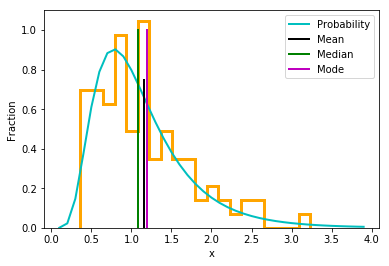In :
import numpy as np
import matplotlib.pyplot as plt
%matplotlib inline
from IPython.display import Image
import pandas as pd


### Lecture 15:¶

• Learn some basic statisics - samples versus populations and empirical versus theorectical distributions.

• Learn to calculate central tendencies, spreads.

• Learn some useful functions in NumPy and SciPy for simulating distributions and calculating statistics.

## Basic statistical concepts¶

Much of this lecture has been cribbed from the open source paper by Olea (2008) available in its entirety here: https://pubs.usgs.gov/of/2008/1017/ofr2008-1017_rev.pdf which in turn was cribbed from Davis, J. (2002: Statistical and Data Analysis in Geology, 3rd Ed, Wiley, Hoboken).

While this is not a class in statistics, many Earth Scientists write programs to calculate statistics, evaluate significance, and estimate averages and their uncertainties.

So, what is (are?) statistics? Statistics is the way we analyze, interpret and model data. To do it properly we need to understand a few concepts:

1) accuracy versus precision: accuracy is how close your data are to the "truth" while precision is the reproducibility of your data.

2) population versus sample: the population is the set of all possible outcomes of a given measurement (if you had an infinite number of data points), while the sample is what you have - a finite number of data points.

3) probability: Probability is the measure of how likely it is for a particular event to occur. If something is impossible, it has a probability ($P$) of 0. If it is a certainty, it has a probability $P$ of 1.

4) Theoretical versus empirical distributions: Empirical distributions are measured data. Theoretical distributions are analytical probabililty functions (mathematical equations) that can be described with an equation. These can be applied to data, allowing interpretations about the likelihood of observing a given measurement, whether sets of data are "different" from theoretical or other empirical data sets and other powerful statistical tests.

Now we will go through each of these concepts in a bit more detail.

## Accuracy versus precision¶

In :
Image(filename='Figures/accuracy_precision.png',width=700)

Out: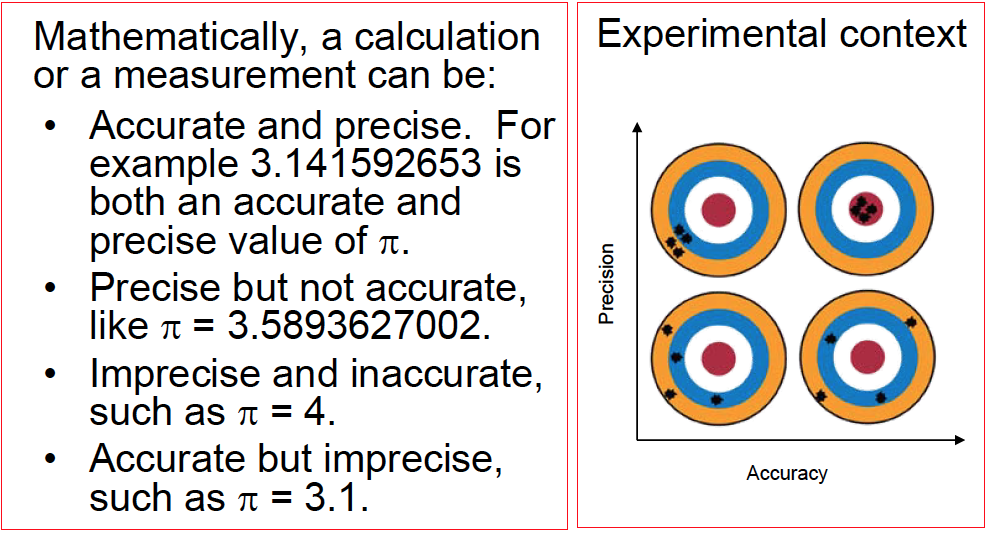## Populations versus samples¶

The population is what you would have if you had all possible outcomes - but you never do. We can describe the distribution of a population by using equations which will predict, say, the fraction of measurements (the density) expected to fall within a given range ($x$ between 0 and 1), assuming a particular theoretical distribution. This curve is called the probability density function.

There are equations describing many different types of distributions and evaluating the equations gives us a theoretical distribution. In this lecture, we will look at a few common distributions (binomial, uniform, normal, and log-normal).

Samples are finite collections of observations which may belong to a given distribution. In this lecture, it will be handy to simulate 'measurements' by drawing 'observations' from a theoretical distribution, instead of making actual measurements. This is the Monte Carlo approach (after the gambling town).

Examples of theoretical versus empirical distributions:

### Binomial distribution:¶

#### Theoretical¶

Perhaps the most straight forward distribution is the binomial distribution which describes the probability of a particular outcome when there are only two possibilities (yes or no, heads or tails, 1 or 0). For example, in a coin toss experiment (heads or tails), if we flip the coin $n$ times, what is the probability of getting $x$ 'heads'? We assume that the probability $p$ of a head for any given coin toss is 50%; put another way $p$ = 0.5.

The binomial distribution can be described by an equation:

$$P=f(x,p,n)= \frac{n!}{x!(n-x)!}p^x(1-p)^{n-x}, x=0,1,2,...,n.$$

We can look at this kind of distribution by evaluating the probability for getting $x$ 'heads' out of $n$ attempts using our old friend from Lecture 11, the lambda function. We'll code the equation as a lambda function, and calculate the probability $P$ of a particular outcome (e.g., $x$ heads in $n$ attempts). We can collect all the answers in a list and plot the probability ($P$) versus the number of heads ($x$) out of $n$ attempts.

Here is a lambda function called Binomial, which returns the probability of a given number of heads ($x$) out of $n$ attempts. Note that for a coin toss, $p$ is 0.5, but other yes/no questions can be investigated as well (e.g., chance of winning the lottery given purchase of $n$ tickets). Oh and we finally have a use for the np.factorial( ) function we ran accross in Lecture 11. Or you could our own reduce funtion. :)

In :
Binomial=lambda x,n,p :(np.math.factorial(n)/(np.math.factorial(x)*np.math.factorial(n-x)))\
*(p**(x))*(1.-p)**(n-x)



We can use Binomial to look at the predicted likelihood of getting $x$ heads out of $n=12$ attempts (coin tosses) with a $p$ (probability) of 0.5.

In :
 # calculate the probability  of x heads in n attempts with a probability of 0.5
n,p=12,0.5 # number of attempts in each trial, probability of getting a head
xs=range(n+1) # range of test values from 0,N
Probability=[] # probability of getting x heads out of n attempts
for x in xs: # step trough the test values
Probability.append(Binomial(x,n,p))# collect the theoretical probability of getting x heads
plt.bar(xs,Probability,width=.5,color='cyan') # plot as bar plot
plt.plot(xs,Probability,'r-',linewidth=2) # plot as solid line
plt.xlabel('Number of heads out of $n$ attempts') # add labels
plt.ylabel('Probability')
# place a note in upper left in axes coordinates with a fontsize of 14.
plt.text(1,.2, 'n = %i'%(n),  fontsize=14)
plt.title('Binomial Distribution');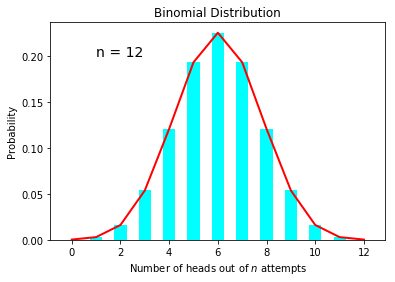Red line is the theoretical probability distribution function. Cyan bars are the same, but plotted as a bar graph.

I plotted the outcome as both a bar plot and a solid red line, to demonstrate two different ways of visualizing the results. Note that the red line is the probability density function.

What you learn from this is that the most probable outcome is 6 out of 12 heads (with a $P$ of ~23%), but other outcomes can very well occur.

#### Empirical¶

One great feature about computers is that we can simulate a data sample to compare to our theoretical predictions. We can use the module numpy.random to generate examples of simulated data sets in a process called Monte Carlo simulation. We encountered numpy.random( ) in the Lecture 14 when we used the random.randint( ) function. In this lecture we will discover a few more, starting with random.binomial( ) which generates samples from a binomial distribution.

To use all the functions in random we must first import it.

In :
from numpy import random
help(random.binomial)

Help on built-in function binomial:

binomial(...) method of mtrand.RandomState instance
binomial(n, p, size=None)

Draw samples from a binomial distribution.

Samples are drawn from a binomial distribution with specified
parameters, n trials and p probability of success where
n an integer >= 0 and p is in the interval [0,1]. (n may be
input as a float, but it is truncated to an integer in use)

Parameters
----------
n : int or array_like of ints
Parameter of the distribution, >= 0. Floats are also accepted,
but they will be truncated to integers.
p : float or array_like of floats
Parameter of the distribution, >= 0 and <=1.
size : int or tuple of ints, optional
Output shape.  If the given shape is, e.g., (m, n, k), then
m * n * k samples are drawn.  If size is None (default),
a single value is returned if n and p are both scalars.
Otherwise, np.broadcast(n, p).size samples are drawn.

Returns
-------
out : ndarray or scalar
Drawn samples from the parameterized binomial distribution, where
each sample is equal to the number of successes over the n trials.

--------
scipy.stats.binom : probability density function, distribution or
cumulative density function, etc.

Notes
-----
The probability density for the binomial distribution is

.. math:: P(N) = \binom{n}{N}p^N(1-p)^{n-N},

where :math:n is the number of trials, :math:p is the probability
of success, and :math:N is the number of successes.

When estimating the standard error of a proportion in a population by
using a random sample, the normal distribution works well unless the
product p*n <=5, where p = population proportion estimate, and n =
number of samples, in which case the binomial distribution is used
instead. For example, a sample of 15 people shows 4 who are left
handed, and 11 who are right handed. Then p = 4/15 = 27%. 0.27*15 = 4,
so the binomial distribution should be used in this case.

References
----------
..  Dalgaard, Peter, "Introductory Statistics with R",
Springer-Verlag, 2002.
..  Glantz, Stanton A. "Primer of Biostatistics.", McGraw-Hill,
Fifth Edition, 2002.
..  Lentner, Marvin, "Elementary Applied Statistics", Bogden
and Quigley, 1972.
..  Weisstein, Eric W. "Binomial Distribution." From MathWorld--A
Wolfram Web Resource.
http://mathworld.wolfram.com/BinomialDistribution.html
..  Wikipedia, "Binomial distribution",
http://en.wikipedia.org/wiki/Binomial_distribution

Examples
--------
Draw samples from the distribution:

>>> n, p = 10, .5  # number of trials, probability of each trial
>>> s = np.random.binomial(n, p, 1000)
# result of flipping a coin 10 times, tested 1000 times.

A real world example. A company drills 9 wild-cat oil exploration
wells, each with an estimated probability of success of 0.1. All nine
wells fail. What is the probability of that happening?

Let's do 20,000 trials of the model, and count the number that
generate zero positive results.

>>> sum(np.random.binomial(9, 0.1, 20000) == 0)/20000.
# answer = 0.38885, or 38%.



To generate some data, you could either patiently do the experiment with a coin toss, or we can just use the random.binomial function to simulate 'realistic' data.

random.binomial( ) requires 2 parameters, $n$ and $p$, with an optional keyword argument size (if size is not specified, it returns a single trial). Each call to random.binomial( ) returns the number of heads flipped in a single trial of $n$ coin tosses, given the probablity $p=0.5$.

Let's try it with $n=12$ and $p=0.5$. This little code block returns the number of heads out of $n$ attempts. You will get a different answer every time you run it. It also gives the probabilty of getting that result from our lambda function Binomial( ).

In :
n,p=12,0.5 # same as before
x=random.binomial(n,p) # size = 1 by default
print (x, 'heads, with a likelihood of: ',Binomial(x,n,p))

7 heads, with a likelihood of:  0.193359375


As the number of times you repeat this 'experiment' approaches infinity, the distribution of outcomes will approach the theoretical distribution (i.e., you will get an average of 9 heads out of 12 attempts 5% of the time).

So let's compare the results simulated via Monte Carlo for some number of experiments ($Nmc$) with the theoretical distribution. To do this, we pretend that each student in the class ($Nmc=20$) flips a coin $n=12$ times and reports the number of heads. We can collect the number of heads flipped by each student in a list called Simulated.

In :
n,p=12,0.5
Nmc=20 # number of simulated experiments each with n  attempts
Simulated=random.binomial(n,p,size=Nmc)
plt.bar(xs,Probability,color='cyan', label='Theoretical') # theoretical curve as bar graph
plt.hist(Simulated,density=True,color='orange',histtype='step',linewidth=3, label='Simulated') # note the normed key word -
#  this normalizes the total to be unity
plt.xlabel('Number of heads out of $n$ attempts')
plt.ylabel('Fraction of simulated experiments')
plt.text(1,.3, 'n = %i'%(n),  fontsize=14)
plt.legend();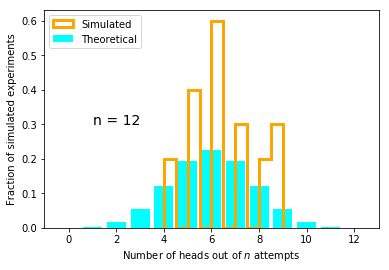Orange line is the Monte Carlo results. Blue line is the theoretical probability distribution function from before.

Notice that every time you repeat this, the Monte Carlo results are a little different. And if you change $Nmc$ to be, say 10, you get more and more "weird" results. But if you set $Nmc$ to 5000 - your results would look consistently closer to the theoretical predictions.

It is worth pointing out here that it is possible to make sure that every time you repeat a "random draw" that you get the SAME answer (for reproducibility of your code, for example if you share it with others). To do this you can use the random.random(seed) option where $seed$ is set in your program. Here is examples with and without the seed:

In :
seed=2019
print ('random numbers generated with seed')
for i in range(10):
random.seed(seed)
print (random.binomial(n,p,size=Nmc))
print ('random numbers generated without seed')
for i in range(10):
print (random.binomial(n,p,size=Nmc))

random numbers generated with seed
[ 8  6  7  7  8  5  7  8  8  6  6  5  4  8  4 10  3  6  5  8]
[ 8  6  7  7  8  5  7  8  8  6  6  5  4  8  4 10  3  6  5  8]
[ 8  6  7  7  8  5  7  8  8  6  6  5  4  8  4 10  3  6  5  8]
[ 8  6  7  7  8  5  7  8  8  6  6  5  4  8  4 10  3  6  5  8]
[ 8  6  7  7  8  5  7  8  8  6  6  5  4  8  4 10  3  6  5  8]
[ 8  6  7  7  8  5  7  8  8  6  6  5  4  8  4 10  3  6  5  8]
[ 8  6  7  7  8  5  7  8  8  6  6  5  4  8  4 10  3  6  5  8]
[ 8  6  7  7  8  5  7  8  8  6  6  5  4  8  4 10  3  6  5  8]
[ 8  6  7  7  8  5  7  8  8  6  6  5  4  8  4 10  3  6  5  8]
[ 8  6  7  7  8  5  7  8  8  6  6  5  4  8  4 10  3  6  5  8]
random numbers generated without seed
[6 6 5 8 6 4 5 3 6 6 8 9 7 8 9 8 8 7 4 5]
[5 4 6 8 5 5 7 4 6 8 5 5 5 5 5 9 3 7 5 7]
[6 7 5 7 5 5 8 8 6 7 5 5 6 6 8 7 9 5 6 5]
[5 5 5 6 6 7 4 5 6 6 2 7 7 9 6 6 7 6 5 3]
[ 4  7  5  7  7  4  9  8  5  5  4  7  3  5  3 10  4  4  5  6]
[5 9 5 6 5 4 9 7 8 5 3 6 3 3 7 6 7 9 5 5]
[8 7 8 6 7 4 6 6 5 2 4 4 6 8 7 7 5 9 3 5]
[6 6 4 7 6 3 6 6 3 8 6 6 4 4 8 5 6 7 4 6]
[ 9  8  6  6 10  4  5  9 10  2  3  4  8  5  7  4  8  4  6  7]
[6 6 5 7 7 6 9 6 7 6 6 9 9 6 8 7 5 6 6 4]


For the purposes of this lecture, we want different random numbers every time, so we will work without the seed. Also, something about the 'density' (formerly 'normed') keyword in plt.hist( ). According to the NumPy documention for density: If it is set to 'True', the result is the value of the probability density function at the bin level, normalized such that the integral over the entire range is 1. But note: the sum of the histogram values will not equal 1 unless bins of unity width are used.

There are many other distributions that we could play with. In the rest of the lecture, we will look at a few of the more common ones in Earth Science: uniform, normal and log normal distributions. We'll approach each in the same way as for the binomial distributions starting with the theoretical distribution followed by a Monte Carlo simulation of empirical results.

### Uniform distribution¶

#### Theoretical¶

A uniform distribution is pretty much what it sounds like. All outcomes within the bounds of $a,b$ are equally likely. Outside those bounds, the probability is zero. For example, when playing dice, what is the likelihood of getting a particular number of dots (1 in 6).

The analytical form for a uniform distribution is:

$$P=f(x,a,b)= \frac{1}{b-a}, x=a \rightarrow b$$

where $a$ and $b$ are bounds ($a \le x<b$). $P$ is the probability of getting a value of $x$. It is zero if $x$ is not between $a$ and $b$, and between $a$ and $b$ the probability is a constant. In fact the probability is $1/n$ where $n$ is $b-a$, the number of possible outcomes.

Say we have a die with 1 to 6 dots on each face. The probability of getting one dot is 1 in 6, but getting seven dots is zero.

We code up the lambda function as before:

In :
Uniform=lambda a,b : (1./(b-a)) # function for calculating P from a uniform distribution.


And calculate the probability density function as before:

In :
# calculate the probability of returning a value x with a uniform distribution between a,b
xs=range(11) # range of possible number of dots from 1 to 10.
a,b=1,7 # bounds for the uniform distribution
Probability=[] # container for the theoretical results.
for x in xs: # step through test values
if x not in range(a,b): # if x<a or x>b,  there is zero probability of rolling this number
Probability.append(0) # save the probability
else: # otherwise
Probability.append(Uniform(a,b)) # get the probability from our little function
plt.bar(xs,Probability,color='cyan') # plot as a bar plot
plt.title('Uniform')
plt.xlabel('Number of dots')
plt.ylabel('Probability');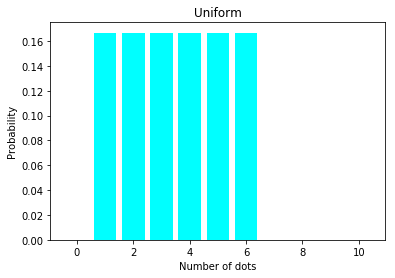#### Empirical¶

And now we can look at the Monte Carlo simulation of an empirical distribution using random.uniform( ).

So here it is.

In :
a,b=1,7 # keep the same bounds
Nmc=20 # number of "students" rolling the dice
Simulated=random.uniform(a,b,Nmc).astype('int') # get Nmc test values in one go.  :)
# the .astype(int) makes this an array of integers, as only integers are possible outcomes
plt.bar(xs,Probability, color='cyan',label='Theoretical')
# plot results as histogram normed to sum to unity and use histtype of 'step' to make it see-through
plt.hist(Simulated,density=True,histtype='step',color='orange',linewidth=3.,label='Simulated')
plt.xlabel('Number of dots')
plt.ylabel('Fraction')
plt.legend();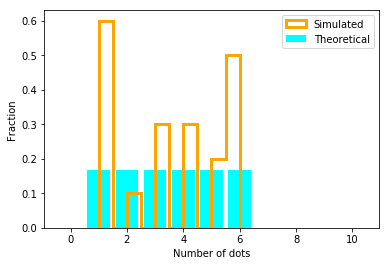There are two more closely related distributions which we need to discuss: the normal and the log-normal distributions. We'll do these with the same approach as before, looking first at the theoretical probability function and then as a Monte Carlo simulation.

### Normal distributions¶

The so-called "normal" distribution (also known as a Gaussian distribution after the guy who thought it up) describes data, for example, measurement data, that have uncertainties associated with them - they are more or less precise. There is some 'true' answer but all the measurements have some slop. Imagine measuring the width of a sedimentary bed, or the length of a fossil thigh bone or the distance between two points. The measurement data will have some average (a.k.a. central tendency) and some degree of spread. For normal distributions, the average is the arithmetic mean $\mu$ and the spread is the standard deviation, $\sigma$.

#### Theoretical:¶

The analytical form for a normal distribution is:

$$P=f(x,\mu, \sigma)= \frac{1}{\sigma\sqrt{2\pi}} e^{-\frac{(x-\mu)^2}{2\sigma^2}},-\infty < x < \infty$$

We can put this equation into a lambda function called Normal and work on it as before with one small modification. We can write the Normal lambda function such that it returns an array if $x$ is passed as an array. This is a trick called 'vectorization' and is numerically more efficient than calling the function many times and appending to a list of results, for example. Use this trick any time you can!

In :
Normal=lambda x,mu,sigma : (1./(sigma*np.sqrt(2.**np.pi)))*np.e**(-(x-mu)**2/(2.*sigma**2))


And, we calculate the probability of observing a measurement $x$ from a normal distribution with $\mu$ and $\sigma$.

In :
mu,sigma,incr=10,1,.2 # set the mean,  standard deviation and bin width
xs=np.arange(5,15,incr) # make an array of test values
Probability=Normal(xs,mu,sigma) # get probabilities
plt.bar(xs,Probability,width=incr,color='cyan', edgecolor='k') # make the bar chart
plt.plot(xs,Probability,'r-',linewidth=2) # plot as a continuous probability distribution
plt.xlabel('x')
plt.ylabel('Probability')
plt.text(5,.3,'$\mu$ = '+str(mu)) # stick on some notes
plt.text(5,.27,'$\sigma$ ='+str(sigma))
plt.title('Normal');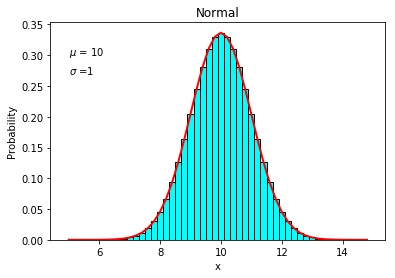That should look a lot like the so-called 'bell curve' - the curve used for grading for example - because that is exactly what it is.

#### Empirical¶

So let's compare the bell curve with some simulated data. For this, we can use random.normal( ).

In :
mu,sigma=10,1 # set the mean,  standard deviation
Nmc=20 # number of monte carlo simultions
Simulated=random.normal(mu,sigma,Nmc) # get Nmc  simulated data points from distribution
plt.bar(xs,Probability,width=incr, edgecolor='k',label='Theoretical',color='cyan') # make the bar chart
plt.hist(Simulated,density=True,histtype='step',color='orange',linewidth=3.,label='Simulated') # plot them
plt.legend();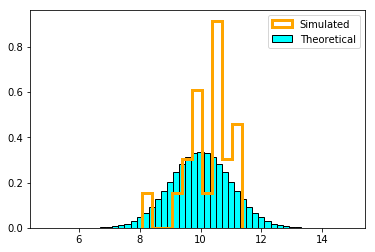We talked about the mean and standard deviation of a theoretical distribution. Now we need to learn how to calculate them for our simulated sample (in case you don't know already!). Note that for the population (as defined above), the average is $\mu$ but for our sample, it is $\bar x$:

$$\bar{x} = \frac{\sum x_i}{N},$$

where $\sum_{i=1}^N$ means the sum of all individual values of $x$ from the first to the last.

Similarly, the standard deviation of the population is $\sigma$, while for our sample, it is $s$:

$$s = \sqrt {\frac {1}{N}\sum_{i=1}^{N}(x_i-\bar x)^2 }$$

Note that the variance of the data is $s^2$ (and for the population it is $\sigma^2$).

With NumPy, we can calculate the mean and standard deviation with the methods .mean( ) and .std( ) like this:

In :
print ('Mean of the simulated distribution = ',Simulated.mean())
print ('standard deviation of simulated distribution =',Simulated.std())

Mean of the simulated distribution =  10.086868749412135
standard deviation of simulated distribution = 0.8782405911779055


Now we need to remember about formating and significant figures from a few lectures ago. For our purposes, only the first few of these trailing decimals are significant, so we should format our print string accordingly. These are floating point variables and say we want a total of up to, say, 6 characters with two after the decimal point. We would write this with a string formatting statement with the form '%XXX.XXf' %(variable).

In :
print ('Mean of the simulated distribution = %6.2f'%(Simulated.mean() ))
print ('standard deviation of simulated distribution =','%6.2f'%(Simulated.std() ))

Mean of the simulated distribution =  10.09
standard deviation of simulated distribution =   0.88


Let's plot the mean and $s$ on our histogram:

In :
plt.bar(xs,Probability,width=incr,color='cyan',label='Theoretical') # make the bar chart
#stderr=Simulated.std()/np.sqrt(len(Simulated))
plt.hist(Simulated,density=True,histtype='step',color='orange',linewidth=3.,label='Simulated') # plot them
plt.plot([Simulated.mean(),Simulated.mean()],[0,.45],'k-',linewidth=2,label='Mean')
plt.plot([Simulated.mean()-Simulated.std(),Simulated.mean()-Simulated.std()],[0,.45],
'g--',linewidth=2,label='Standard deviation')
plt.plot([Simulated.mean()+Simulated.std(),Simulated.mean()+Simulated.std()],[0,.45],
'g--',linewidth=2,label='_nolegend_') # notice how to suppress a legend entry!
plt.ylim(0,.5)
plt.legend(loc=2);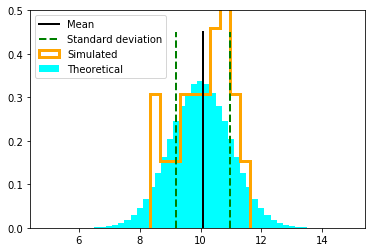Notice two things:

1) The standard deviation includes ~67% of the data (not 95% - that would be 1.97$\sigma$, or 2-sigma, informally). The $\pm \sigma$ bounds are the dashed lines in the above plot.

2) The mean of our sample is generally not the same as the mean of the distribution ($\bar x \ne \mu$). In fact, the 95% confidence bounds for the MEAN is related to the 'standard error', which is:

$s_e = \frac {s}{\sqrt N}$.

The 95% confidence bounds for the mean is given by 1.97$s_e$. This means in practice that the mean will be more than 1.97$s_e$ away from true mean 5% of the time. We could test that statement with another little Monte Carlo type simulation, but I leave that to student curiosity.

Now we need to look at one last distribution: the log-normal distribution.

### Log normal¶

Many things in nature are not normally distributed. Many processes lead to distributions in which the log of the quantity is normally distributed instead of the quantity itself. For example, grain sizes in sedimentary sequences are often log normally distributed. These distributions are log-normal and behave differently than normal distributions.

#### Theoretical¶

The equation for the log-normal probability density function is this:

$$P=f(x,\mu, \sigma)= \frac{1}{x\sigma \sqrt{2\pi}} \exp {\bigl(-\frac{(\text{ln}(x)-\mu)^2}{2\sigma^2}}\bigr),0< x < \infty$$

Notice that $x$ is in the denominator so we can't evaluate the probability when $x$=0.

As before we make a lambda function to evaluate the probability density of log-normal distributions:

In :
# Here is a log normal probability density function maker:
LogNormal=lambda x,mu,sigma : (1./(x*sigma*np.sqrt(2.*np.pi)))*np.e**(-((np.log(x)-mu)**2)/(2.*sigma**2))


And we evaluate it for a range of possible values of $x$ for given $\mu, \sigma$.

In :
# calculate the probability of returning a value x with a normal distribution with mu and sigma
mu,sigma,incr=0,.5,.1 # set the mean,  standard deviation and bin width
xs=np.arange(incr,4,incr) # make an array of test values
Probability=LogNormal(xs,mu,sigma) # get probabilities for the array of Xs
plt.bar(xs,Probability,width=incr,color='cyan', edgecolor='k') # make the bar chart
plt.plot(xs,Probability,'r-',linewidth=2) # plot as a continuous probability distribution
plt.title('Log Normal Distribution')
plt.xlabel('X')
plt.ylabel('Probability');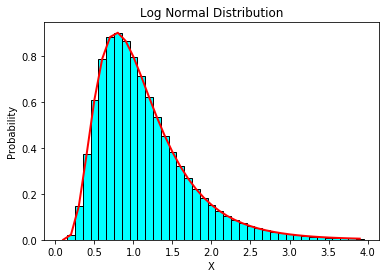#### Empirical¶

To simulate data, we can use random.lognormal( ), specifying mean and standard deviation as for the normal distribution.

Here we plot the theoretical distribution and the simulated data, along with its mean and the $\pm \sigma$ bounds calculated as we did in the last lecture for normal distributions.

In :
mu,sigma=0,.5 # set the mean,  standard deviation
Nmc=100 # number of monte carlo simultions
Simulated=random.lognormal(mu,sigma,Nmc) # get Nmc  simulated data points from distribution
plt.bar(xs,Probability,width=incr,color='cyan',edgecolor='k',label='Theoretical') # make the bar chart
plt.hist(Simulated,density=True,histtype='step',color='orange',linewidth=3.,label='Simulated') # plot them
plt.plot([Simulated.mean(),Simulated.mean()],[0,1],'k-',linewidth=2,label='Mean')
plt.plot([Simulated.mean()-Simulated.std(),Simulated.mean()-Simulated.std()],[0,1],
'g--',linewidth=2,label='lower bound')
plt.plot([Simulated.mean()+Simulated.std(),Simulated.mean()+Simulated.std()],[0,1],
'r--',linewidth=2,label='upper bound')
plt.xlabel('x')
plt.ylabel('Frequency')
plt.legend();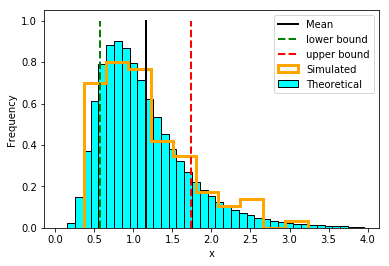### Hey!¶

Why is the mean way off to the right? And those confidence bounds don't look right at all!

It turns out that the mean as defined in the last lecture is only good for 'normal' distributions and shouldn't be used for other types.

First, let's look at the plot using the log normal of the data instead of the data themselves:

In :
barx=np.log(Simulated).mean() # get the  mean of the logs of the  simulated data set
s=np.log(Simulated).std() # get the standard deviation of same
plt.hist(np.log(Simulated),density=True,histtype='step',color='orange',linewidth=3) # plot them
plt.plot([barx,barx],\
[0,1],'k-',linewidth=2);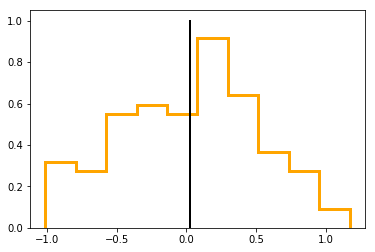It appears that a log normal distribution looks more "normal" when plotted on a log scale. But the mean doesn't behave the way we expect for normal distributions (offset to the long tail).

So what would be a sensible way of describing the central tendency (Expectation $E$) and spread (variance) for a log normal distribution?

These are expressed as follows:

$$E(x) =\exp\bigr( \mu +\frac{1}{2}\sigma^2 \bigl),$$

and

$$\text{var}(x) = \bigr[\exp (\sigma^2)-1\bigr]*\exp(2\mu + \sigma^2)$$

where $\mu, \sigma$ are the mean and standard deviation of the logs of the population. For the sample, we will refer to them at $\bar x$ and $s$ respectively and for the expectation and variance we will use $m$ and $v$.

Fortunately, there is a nice SciPy package that does statistics for log normal distributions. SciPy is a very powerful package of scientific python functions, many of which were later incorporated into NumPy, but not all. One of the "leftovers" is scipy.stats.lognorm. You can take a look at what lognorm can do for us using help(stats.lognorm) after we import it, if you are curious.

In :
from scipy import stats


Ok - you should be curious! So let's take a look at the help message:

In :
help(stats.lognorm)

Help on lognorm_gen in module scipy.stats._continuous_distns object:

class lognorm_gen(scipy.stats._distn_infrastructure.rv_continuous)
|  A lognormal continuous random variable.
|
|  %(before_notes)s
|
|  Notes
|  -----
|  The probability density function for lognorm is:
|
|  .. math::
|
|      f(x, s) = \frac{1}{s x \sqrt{2\pi}}
|                \exp(-\frac{1}{2} (\frac{\log(x)}{s})^2)
|
|  for x > 0, s > 0.
|
|  lognorm takes s as a shape parameter.
|
|  %(after_notes)s
|
|  A common parametrization for a lognormal random variable Y is in
|  terms of the mean, mu, and standard deviation, sigma, of the
|  unique normally distributed random variable X such that exp(X) = Y.
|  This parametrization corresponds to setting s = sigma and scale =
|  exp(mu).
|
|  %(example)s
|
|  Method resolution order:
|      lognorm_gen
|      scipy.stats._distn_infrastructure.rv_continuous
|      scipy.stats._distn_infrastructure.rv_generic
|      builtins.object
|
|  Methods defined here:
|
|  fit(self, data, *args, **kwds)
|      Return MLEs for shape (if applicable), location, and scale
|      parameters from data.
|
|      MLE stands for Maximum Likelihood Estimate.  Starting estimates for
|      the fit are given by input arguments; for any arguments not provided
|      with starting estimates, self._fitstart(data) is called to generate
|      such.
|
|      One can hold some parameters fixed to specific values by passing in
|      keyword arguments f0, f1, ..., fn (for shape parameters)
|      and floc and fscale (for location and scale parameters,
|      respectively).
|
|      Parameters
|      ----------
|      data : array_like
|          Data to use in calculating the MLEs.
|      args : floats, optional
|          Starting value(s) for any shape-characterizing arguments (those not
|          provided will be determined by a call to _fitstart(data)).
|          No default value.
|      kwds : floats, optional
|          Starting values for the location and scale parameters; no default.
|          Special keyword arguments are recognized as holding certain
|          parameters fixed:
|
|          - f0...fn : hold respective shape parameters fixed.
|            Alternatively, shape parameters to fix can be specified by name.
|            For example, if self.shapes == "a, b", faand fix_a
|            are equivalent to f0, and fb and fix_b are
|            equivalent to f1.
|
|          - floc : hold location parameter fixed to specified value.
|
|          - fscale : hold scale parameter fixed to specified value.
|
|          - optimizer : The optimizer to use.  The optimizer must take func,
|            and starting position as the first two arguments,
|            plus args (for extra arguments to pass to the
|            function to be optimized) and disp=0 to suppress
|            output as keyword arguments.
|
|      Returns
|      -------
|      mle_tuple : tuple of floats
|          MLEs for any shape parameters (if applicable), followed by those
|          for location and scale. For most random variables, shape statistics
|          will be returned, but there are exceptions (e.g. norm).
|
|      Notes
|      -----
|      This fit is computed by maximizing a log-likelihood function, with
|      penalty applied for samples outside of range of the distribution. The
|      returned answer is not guaranteed to be the globally optimal MLE, it
|      may only be locally optimal, or the optimization may fail altogether.
|
|      When the location parameter is fixed by using the floc argument,
|      this function uses explicit formulas for the maximum likelihood
|      estimation of the log-normal shape and scale parameters, so the
|      optimizer, loc and scale keyword arguments are ignored.
|
|      Examples
|      --------
|
|      Generate some data to fit: draw random variates from the beta
|      distribution
|
|      >>> from scipy.stats import beta
|      >>> a, b = 1., 2.
|      >>> x = beta.rvs(a, b, size=1000)
|
|      Now we can fit all four parameters (a, b, loc and scale):
|
|      >>> a1, b1, loc1, scale1 = beta.fit(x)
|
|      We can also use some prior knowledge about the dataset: let's keep
|      loc and scale fixed:
|
|      >>> a1, b1, loc1, scale1 = beta.fit(x, floc=0, fscale=1)
|      >>> loc1, scale1
|      (0, 1)
|
|      We can also keep shape parameters fixed by using f-keywords. To
|      keep the zero-th shape parameter a equal 1, use f0=1 or,
|      equivalently, fa=1:
|
|      >>> a1, b1, loc1, scale1 = beta.fit(x, fa=1, floc=0, fscale=1)
|      >>> a1
|      1
|
|      Not all distributions return estimates for the shape parameters.
|      norm for example just returns estimates for location and scale:
|
|      >>> from scipy.stats import norm
|      >>> x = norm.rvs(a, b, size=1000, random_state=123)
|      >>> loc1, scale1 = norm.fit(x)
|      >>> loc1, scale1
|      (0.92087172783841631, 2.0015750750324668)
|
|  ----------------------------------------------------------------------
|  Methods inherited from scipy.stats._distn_infrastructure.rv_continuous:
|
|  __init__(self, momtype=1, a=None, b=None, xtol=1e-14, badvalue=None, name=None, longname=None, shapes=None, extradoc=None, seed=None)
|      Initialize self.  See help(type(self)) for accurate signature.
|
|  cdf(self, x, *args, **kwds)
|      Cumulative distribution function of the given RV.
|
|      Parameters
|      ----------
|      x : array_like
|          quantiles
|      arg1, arg2, arg3,... : array_like
|          The shape parameter(s) for the distribution (see docstring of the
|      loc : array_like, optional
|          location parameter (default=0)
|      scale : array_like, optional
|          scale parameter (default=1)
|
|      Returns
|      -------
|      cdf : ndarray
|          Cumulative distribution function evaluated at x
|
|  expect(self, func=None, args=(), loc=0, scale=1, lb=None, ub=None, conditional=False, **kwds)
|      Calculate expected value of a function with respect to the
|      distribution.
|
|      The expected value of a function f(x) with respect to a
|      distribution dist is defined as::
|
|                  ubound
|          E[x] = Integral(f(x) * dist.pdf(x))
|                  lbound
|
|      Parameters
|      ----------
|      func : callable, optional
|          Function for which integral is calculated. Takes only one argument.
|          The default is the identity mapping f(x) = x.
|      args : tuple, optional
|          Shape parameters of the distribution.
|      loc : float, optional
|          Location parameter (default=0).
|      scale : float, optional
|          Scale parameter (default=1).
|      lb, ub : scalar, optional
|          Lower and upper bound for integration. Default is set to the
|          support of the distribution.
|      conditional : bool, optional
|          If True, the integral is corrected by the conditional probability
|          of the integration interval.  The return value is the expectation
|          of the function, conditional on being in the given interval.
|          Default is False.
|
|      Additional keyword arguments are passed to the integration routine.
|
|      Returns
|      -------
|      expect : float
|          The calculated expected value.
|
|      Notes
|      -----
|      The integration behavior of this function is inherited from
|      integrate.quad.
|
|  fit_loc_scale(self, data, *args)
|      Estimate loc and scale parameters from data using 1st and 2nd moments.
|
|      Parameters
|      ----------
|      data : array_like
|          Data to fit.
|      arg1, arg2, arg3,... : array_like
|          The shape parameter(s) for the distribution (see docstring of the
|
|      Returns
|      -------
|      Lhat : float
|          Estimated location parameter for the data.
|      Shat : float
|          Estimated scale parameter for the data.
|
|  isf(self, q, *args, **kwds)
|      Inverse survival function (inverse of sf) at q of the given RV.
|
|      Parameters
|      ----------
|      q : array_like
|          upper tail probability
|      arg1, arg2, arg3,... : array_like
|          The shape parameter(s) for the distribution (see docstring of the
|      loc : array_like, optional
|          location parameter (default=0)
|      scale : array_like, optional
|          scale parameter (default=1)
|
|      Returns
|      -------
|      x : ndarray or scalar
|          Quantile corresponding to the upper tail probability q.
|
|  logcdf(self, x, *args, **kwds)
|      Log of the cumulative distribution function at x of the given RV.
|
|      Parameters
|      ----------
|      x : array_like
|          quantiles
|      arg1, arg2, arg3,... : array_like
|          The shape parameter(s) for the distribution (see docstring of the
|      loc : array_like, optional
|          location parameter (default=0)
|      scale : array_like, optional
|          scale parameter (default=1)
|
|      Returns
|      -------
|      logcdf : array_like
|          Log of the cumulative distribution function evaluated at x
|
|  logpdf(self, x, *args, **kwds)
|      Log of the probability density function at x of the given RV.
|
|      This uses a more numerically accurate calculation if available.
|
|      Parameters
|      ----------
|      x : array_like
|          quantiles
|      arg1, arg2, arg3,... : array_like
|          The shape parameter(s) for the distribution (see docstring of the
|      loc : array_like, optional
|          location parameter (default=0)
|      scale : array_like, optional
|          scale parameter (default=1)
|
|      Returns
|      -------
|      logpdf : array_like
|          Log of the probability density function evaluated at x
|
|  logsf(self, x, *args, **kwds)
|      Log of the survival function of the given RV.
|
|      Returns the log of the "survival function," defined as (1 - cdf),
|      evaluated at x.
|
|      Parameters
|      ----------
|      x : array_like
|          quantiles
|      arg1, arg2, arg3,... : array_like
|          The shape parameter(s) for the distribution (see docstring of the
|      loc : array_like, optional
|          location parameter (default=0)
|      scale : array_like, optional
|          scale parameter (default=1)
|
|      Returns
|      -------
|      logsf : ndarray
|          Log of the survival function evaluated at x.
|
|  nnlf(self, theta, x)
|      Return negative loglikelihood function.
|
|      Notes
|      -----
|      This is -sum(log pdf(x, theta), axis=0) where theta are the
|      parameters (including loc and scale).
|
|  pdf(self, x, *args, **kwds)
|      Probability density function at x of the given RV.
|
|      Parameters
|      ----------
|      x : array_like
|          quantiles
|      arg1, arg2, arg3,... : array_like
|          The shape parameter(s) for the distribution (see docstring of the
|      loc : array_like, optional
|          location parameter (default=0)
|      scale : array_like, optional
|          scale parameter (default=1)
|
|      Returns
|      -------
|      pdf : ndarray
|          Probability density function evaluated at x
|
|  ppf(self, q, *args, **kwds)
|      Percent point function (inverse of cdf) at q of the given RV.
|
|      Parameters
|      ----------
|      q : array_like
|          lower tail probability
|      arg1, arg2, arg3,... : array_like
|          The shape parameter(s) for the distribution (see docstring of the
|      loc : array_like, optional
|          location parameter (default=0)
|      scale : array_like, optional
|          scale parameter (default=1)
|
|      Returns
|      -------
|      x : array_like
|          quantile corresponding to the lower tail probability q.
|
|  sf(self, x, *args, **kwds)
|      Survival function (1 - cdf) at x of the given RV.
|
|      Parameters
|      ----------
|      x : array_like
|          quantiles
|      arg1, arg2, arg3,... : array_like
|          The shape parameter(s) for the distribution (see docstring of the
|      loc : array_like, optional
|          location parameter (default=0)
|      scale : array_like, optional
|          scale parameter (default=1)
|
|      Returns
|      -------
|      sf : array_like
|          Survival function evaluated at x
|
|  ----------------------------------------------------------------------
|  Methods inherited from scipy.stats._distn_infrastructure.rv_generic:
|
|  __call__(self, *args, **kwds)
|      Freeze the distribution for the given arguments.
|
|      Parameters
|      ----------
|      arg1, arg2, arg3,... : array_like
|          The shape parameter(s) for the distribution.  Should include all
|          the non-optional arguments, may include loc and scale.
|
|      Returns
|      -------
|      rv_frozen : rv_frozen instance
|          The frozen distribution.
|
|  __getstate__(self)
|
|  __setstate__(self, state)
|
|  entropy(self, *args, **kwds)
|      Differential entropy of the RV.
|
|      Parameters
|      ----------
|      arg1, arg2, arg3,... : array_like
|          The shape parameter(s) for the distribution (see docstring of the
|      loc : array_like, optional
|          Location parameter (default=0).
|      scale : array_like, optional  (continuous distributions only).
|          Scale parameter (default=1).
|
|      Notes
|      -----
|      Entropy is defined base e:
|
|      >>> drv = rv_discrete(values=((0, 1), (0.5, 0.5)))
|      >>> np.allclose(drv.entropy(), np.log(2.0))
|      True
|
|  freeze(self, *args, **kwds)
|      Freeze the distribution for the given arguments.
|
|      Parameters
|      ----------
|      arg1, arg2, arg3,... : array_like
|          The shape parameter(s) for the distribution.  Should include all
|          the non-optional arguments, may include loc and scale.
|
|      Returns
|      -------
|      rv_frozen : rv_frozen instance
|          The frozen distribution.
|
|  interval(self, alpha, *args, **kwds)
|      Confidence interval with equal areas around the median.
|
|      Parameters
|      ----------
|      alpha : array_like of float
|          Probability that an rv will be drawn from the returned range.
|          Each value should be in the range [0, 1].
|      arg1, arg2, ... : array_like
|          The shape parameter(s) for the distribution (see docstring of the
|      loc : array_like, optional
|          location parameter, Default is 0.
|      scale : array_like, optional
|          scale parameter, Default is 1.
|
|      Returns
|      -------
|      a, b : ndarray of float
|          end-points of range that contain 100 * alpha % of the rv's
|          possible values.
|
|  mean(self, *args, **kwds)
|      Mean of the distribution.
|
|      Parameters
|      ----------
|      arg1, arg2, arg3,... : array_like
|          The shape parameter(s) for the distribution (see docstring of the
|      loc : array_like, optional
|          location parameter (default=0)
|      scale : array_like, optional
|          scale parameter (default=1)
|
|      Returns
|      -------
|      mean : float
|          the mean of the distribution
|
|  median(self, *args, **kwds)
|      Median of the distribution.
|
|      Parameters
|      ----------
|      arg1, arg2, arg3,... : array_like
|          The shape parameter(s) for the distribution (see docstring of the
|      loc : array_like, optional
|          Location parameter, Default is 0.
|      scale : array_like, optional
|          Scale parameter, Default is 1.
|
|      Returns
|      -------
|      median : float
|          The median of the distribution.
|
|      --------
|      stats.distributions.rv_discrete.ppf
|          Inverse of the CDF
|
|  moment(self, n, *args, **kwds)
|      n-th order non-central moment of distribution.
|
|      Parameters
|      ----------
|      n : int, n >= 1
|          Order of moment.
|      arg1, arg2, arg3,... : float
|          The shape parameter(s) for the distribution (see docstring of the
|      loc : array_like, optional
|          location parameter (default=0)
|      scale : array_like, optional
|          scale parameter (default=1)
|
|  rvs(self, *args, **kwds)
|      Random variates of given type.
|
|      Parameters
|      ----------
|      arg1, arg2, arg3,... : array_like
|          The shape parameter(s) for the distribution (see docstring of the
|      loc : array_like, optional
|          Location parameter (default=0).
|      scale : array_like, optional
|          Scale parameter (default=1).
|      size : int or tuple of ints, optional
|          Defining number of random variates (default is 1).
|      random_state : None or int or np.random.RandomState instance, optional
|          If int or RandomState, use it for drawing the random variates.
|          If None, rely on self.random_state.
|          Default is None.
|
|      Returns
|      -------
|      rvs : ndarray or scalar
|          Random variates of given size.
|
|  stats(self, *args, **kwds)
|      Some statistics of the given RV.
|
|      Parameters
|      ----------
|      arg1, arg2, arg3,... : array_like
|          The shape parameter(s) for the distribution (see docstring of the
|      loc : array_like, optional
|          location parameter (default=0)
|      scale : array_like, optional (continuous RVs only)
|          scale parameter (default=1)
|      moments : str, optional
|          composed of letters ['mvsk'] defining which moments to compute:
|          'm' = mean,
|          'v' = variance,
|          's' = (Fisher's) skew,
|          'k' = (Fisher's) kurtosis.
|          (default is 'mv')
|
|      Returns
|      -------
|      stats : sequence
|          of requested moments.
|
|  std(self, *args, **kwds)
|      Standard deviation of the distribution.
|
|      Parameters
|      ----------
|      arg1, arg2, arg3,... : array_like
|          The shape parameter(s) for the distribution (see docstring of the
|      loc : array_like, optional
|          location parameter (default=0)
|      scale : array_like, optional
|          scale parameter (default=1)
|
|      Returns
|      -------
|      std : float
|          standard deviation of the distribution
|
|  var(self, *args, **kwds)
|      Variance of the distribution.
|
|      Parameters
|      ----------
|      arg1, arg2, arg3,... : array_like
|          The shape parameter(s) for the distribution (see docstring of the
|      loc : array_like, optional
|          location parameter (default=0)
|      scale : array_like, optional
|          scale parameter (default=1)
|
|      Returns
|      -------
|      var : float
|          the variance of the distribution
|
|  ----------------------------------------------------------------------
|  Data descriptors inherited from scipy.stats._distn_infrastructure.rv_generic:
|
|  __dict__
|      dictionary for instance variables (if defined)
|
|  __weakref__
|      list of weak references to the object (if defined)
|
|  random_state
|      Get or set the RandomState object for generating random variates.
|
|      This can be either None or an existing RandomState object.
|
|      If None (or np.random), use the RandomState singleton used by np.random.
|      If already a RandomState instance, use it.
|      If an int, use a new RandomState instance seeded with seed.



The function stats.norm returns 'moments' of data samples, the first two of which are the log normal mean ($m$) and the variance $v$.

So, we should do the following:

• calculate the mean $\bar x$, and standard deviation $s$ of the LOG of the simulated dataset,
• set the scale to be $e^{\bar x}$
• calculate the first few moments, mean ($m$) and variance ($v$).

Now we can calculate the moments, $m$ and $v$ and compare them to the originals $\bar x$ and $s$ and the $\mu$ and $\sigma$ of the theoretical distribution used to generate the simulated data:

In :
m,v=stats.lognorm.stats(s,moments='mv',scale=np.exp(barx))
print ('mu  %5.2f'%(mu),' and barx %5.2f'%(barx))
print ('sigma %5.2f'%(sigma),' and s %5.2f'%(s))
print ('E %5.2f'%(np.exp(mu + 0.5*sigma**2)),' and m %5.2f'%(m))
print ('var %5.3f'%((np.e**(sigma**2)-1)*np.e**(2*mu+sigma**2)),' \
and v  %5.3f'%(v))

mu   0.00  and barx  0.02
sigma  0.50  and s  0.50
E  1.13  and m  1.16
var 0.365         and v  0.379


There is also a NumPy function to calculate the median (np.median( )) and for the mode we can use scipy.stats.mode( ). But be careful with this because it finds the most frequent value, which may have a bunch of significant figures and so each value occurs only once. To get a reasonable estimate of the mode, we first have to round off the numbers in Simulated. We can use np.round() for that.

Here's how to calculate each of these types of expected values:

In :
mean=Simulated.mean() # you knew this already
mean_other_way =np.mean(Simulated) # this is a different way, but works the same.
median=np.median(Simulated) # this is the way to do the median with NumPy
mode,count=stats.mode(np.round(Simulated,1)) # round to 2 significant digits, return mode and number in that mode
print ('mean: ',mean)
print ('mean other way: ',mean_other_way)
print ('median: ',median)
print ('mode: ',mode,' count: ',count)

mean:  1.156629982755693
mean other way:  1.156629982755693
median:  1.0814143428971879
mode:  [1.2]  count:  


Notice that mode and count are both lists.

So let's plot up the data and put the mean, median and the mode on it.

In :
newmode=mode # make this a single number, not a list with one element
plt.hist(Simulated,density=True,bins=20,histtype='step',color='orange',linewidth=3) # plot them
plt.plot(xs,Probability,'c-',linewidth=2,label='Probability'); # plot the theoretical probability distribution function
plt.plot([mean,mean],[0,.75],'k-',linewidth=2,label='Mean') # put on green line, label for legend.
plt.plot([median,median],[0,1],'g-',linewidth=2,label='Median') # black line
plt.plot([newmode,newmode],[0,1],'m-',linewidth=2,label='Mode') # magenta line
plt.legend()
plt.ylim(0,1.1)
plt.xlabel('x'); # set the y axis limits.
plt.ylabel('Fraction');